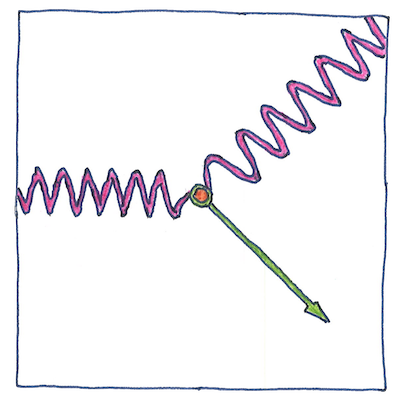# 1923

## The book of science

Tom Sharp

 Arthur Holly Compton physics

## Compton scattering

• When a photon hits an electron,
• the photon transfers energy to the electron,
• is deflected and shifts to a lower wavelength.
• Arthur Holly Compton did the math
• in which each scattered photon
• hits only one electron,
• showing that a photon behaves as a particle,
• not merely as an electromagnetic wave.

## Compton wavelength

• A photon’s energy
• is directly related to its frequency.
• A particle’s mass
• is equivalent to an energy
• by Einstein’s formula, E = mc2.
• The Compton wavelength of a particle
• is the wavelength of a photon
• whose energy is equivalent
• to the particle’s mass.
• The Compton wavelength
• is the amplitude
• of the particle’s
• Zitterbewegung oscillation.

## Inelastic scattering

• When an unstoppable bullet
• hits an impenetrable wall,
• the theory disintegrates.
• There are no such things.
• Let us rephrase the problem.
• Our paths shouldn’t
• depend on which of us
• is the more stubborn.
• No bullet’s unstoppable
• and no wall’s impenetrable.
• Maybe the bullet can turn corners
• and maybe the wall is not complete.
• A little creative thinking,
• a little kindness and respect,
• more altruism and less egotism,
• can stretch the possibilities.

Classical electromagnetic theory predicted that X-rays would not lose energy when scattered. The effect depends on the energy of the photon relative to the energy-equivalent of an electron. Compton scattering is inelastic. It happens when the energy of the photon is higher. Thomson scattering is elastic but happens only when the energy of the photon is much less than the mass energy of the particle (when the particle’s Compton wavelength is much less than the photon’s wavelength).

At the energy of visible light, photons can knock electrons from a surface. This, called the photoelectric effect, supported the concept that energy is quantized.